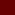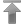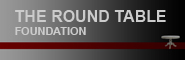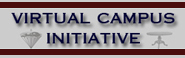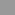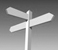Contenido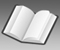Datos# Glosario

 a b c d e f g h i j k l m n o p q r s t u v w x y z

### G

G → Gauss.

Gx, Gy, Gz: conventional symbols for gradient magnetic field. x, y, z denote spatial di­rec­tion component of gradient, i.e. direction along which the field changes.

Gauss (G): a unit of magnetic flux density in the older (CGS) system. The Earth’s mag­ne­tic field is approximately one half gauss to one gauss, depending on location. The pre­fer­red (SI) unit is the Tesla (T) (1 T = 10,000 G = 10 kG).

GE → GRE (preferred); Gradient echo.

Golay coil: term used for a particular kind of gradient coil, commonly used to create gra­di­ent magnetic fields, perpendicular to the main magnetic field.

Gradient: the amount and direction of the rate of change in space of some quantity, such as magnetic field strength.

Gradient coils: current carrying coils designed to produce a desired gradient magnetic field (so that the magnetic field will be stronger in some locations than others). Proper design of the size and configurations of the coils is necessary to produce a controlled and uniform gradient.

Gradient echo: an echo signal generated from an FID by means of a bipolar switched magnetic gradient. Does not refocus the effects of main field inhomogeneity and there­fore is generally used with a short echo time.

Gradient magnetic field: a magnetic field which changes in strength in a certain given direction. Such fields are used in magnetic resonance imaging with selective excitation to select a region for imaging and also to encode the location of NMR signals received from the object being imaged. Measured in milli-Tesla (mT) per meter. Also: magnetic field gradient.

Gradient pulse: briefly applied gradient magnetic field.

GRE → Gradient echo.

Gyromagnetic ratio (γ): the ratio of the magnetic moment to the angular momentum of a particle. This is a constant for a given nucleus.

GRASS → refocused FLASH.

Missing terms? Send us an e-mail. We'll add them ...